# Rosenblatt Transformation¶

The Rosenblatt transformation is an isoprobabilistic transformation (refer to ) which is used under the following context :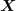is the input random vector,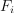the cumulative density functions of its components and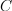its copula, without no condition on its type.
Let us denote by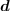a deterministic vector,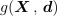the limit state function of the model,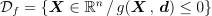the event considered here and g(,) = 0 its boundary.
One way to evaluate the probability content of the event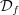:

(1)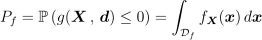is to use the Rosenblatt transformation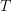which is a diffeomorphism from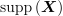into the standard space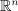, where distributions are normal, with zero mean, unit variance and unit correlation matrix (which is equivalent in that normal case to independent components).

Let us recall some definitions.
The cumulative distribution function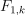of the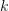-dimensional random vector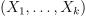is defined by its marginal distributionsand the copula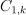through the relation: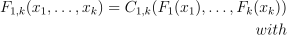(2)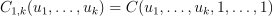The cumulative distribution function of the conditional variable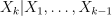is defined by: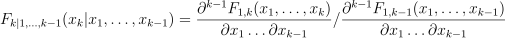Rosenblatt transformation: Letinbe a continuous random vector defined by its marginal cumulative distribution functionsand its copula. The Rosenblatt transformation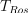ofis defined by:

(3)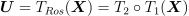where both transformations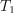, and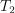are given by:

(4)(5)where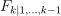is the cumulative distribution function of the conditional random variableand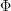the cumulative distribution function of the standard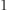-dimensional Normal distribution.